# PHYSICAL QUANTITIES AND MEASUREMENT.pptx

28 de May de 2023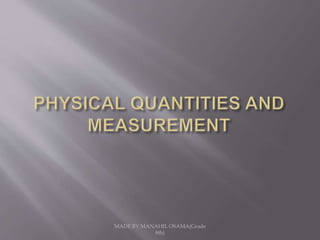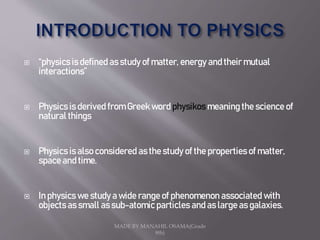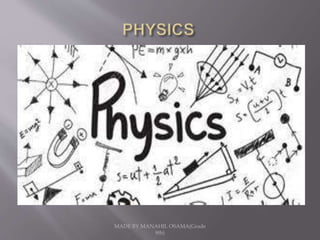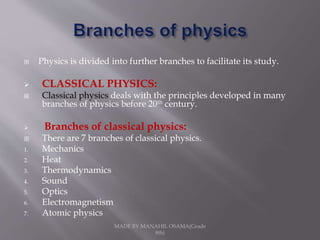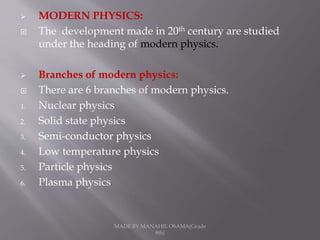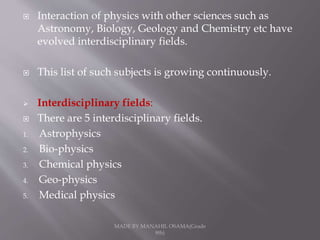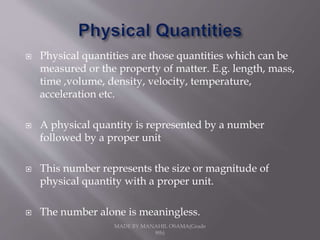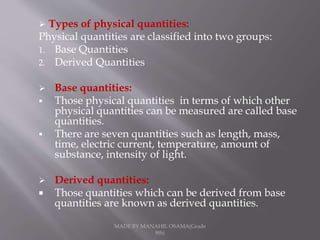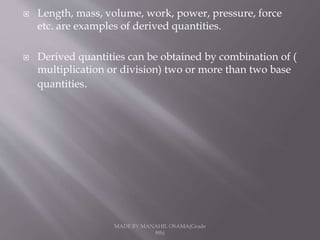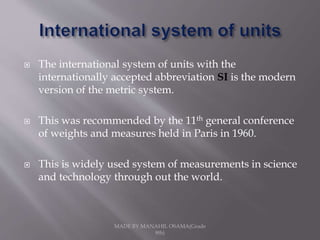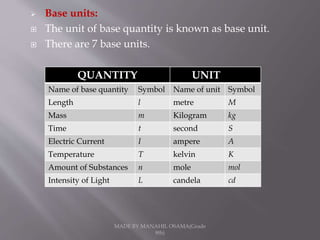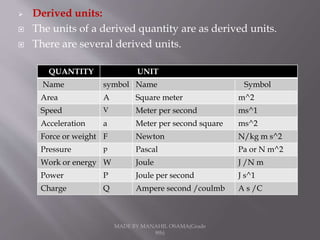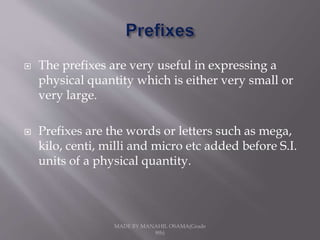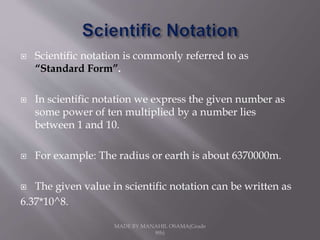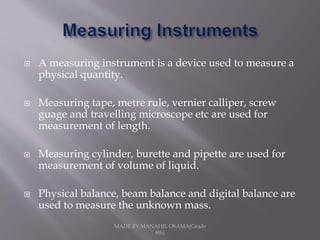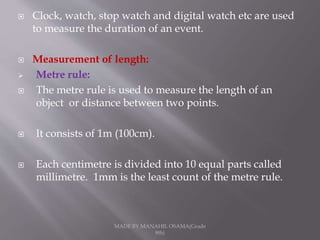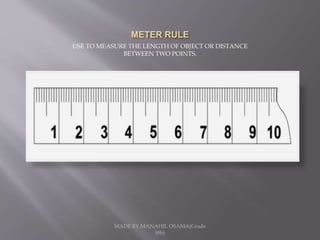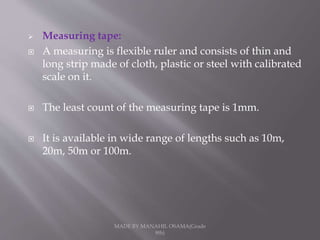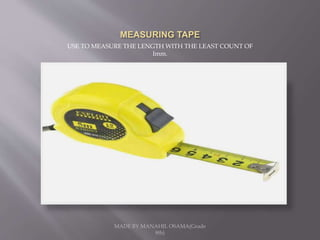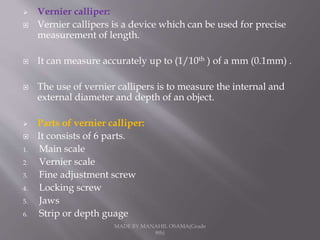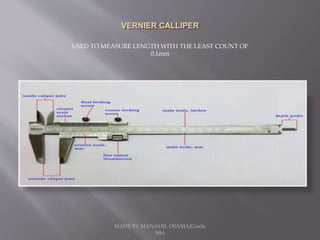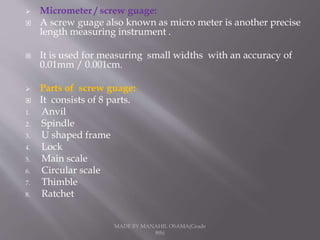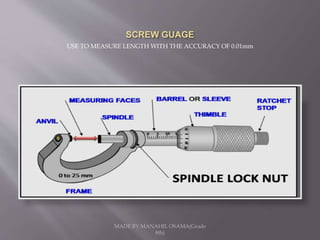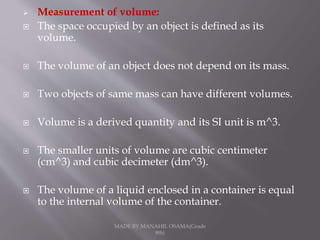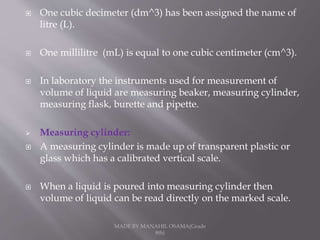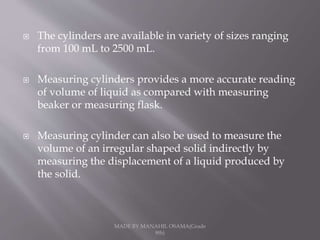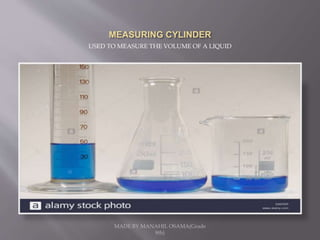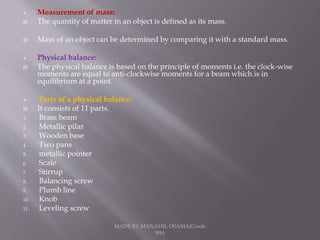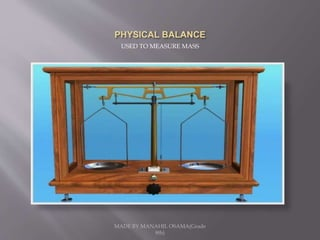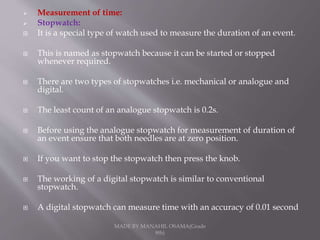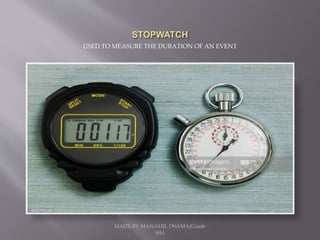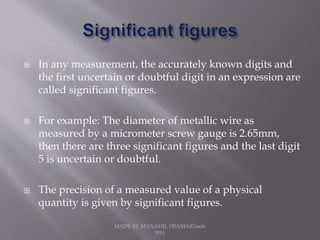1 de 32

### PHYSICAL QUANTITIES AND MEASUREMENT.pptx

• 2.  “physics is defined as study of matter, energy and their mutual interactions”  Physics is derived from Greek word physikos meaning the science of natural things  Physics is also considered as the study of the properties of matter, space and time.  In physics we study a wide range of phenomenon associated with objects as small as sub-atomic particles and as large as galaxies. MADE BY MANAHIL OSAMA(Grade 8th)
• 4.  Physics is divided into further branches to facilitate its study.  CLASSICAL PHYSICS:  Classical physics deals with the principles developed in many branches of physics before 20th century.  Branches of classical physics:  There are 7 branches of classical physics. 1. Mechanics 2. Heat 3. Thermodynamics 4. Sound 5. Optics 6. Electromagnetism 7. Atomic physics MADE BY MANAHIL OSAMA(Grade 8th)
• 5. MADE BY MANAHIL OSAMA(Grade 8th)  MODERN PHYSICS:  The development made in 20th century are studied under the heading of modern physics.  Branches of modern physics:  There are 6 branches of modern physics. 1. Nuclear physics 2. Solid state physics 3. Semi-conductor physics 4. Low temperature physics 5. Particle physics 6. Plasma physics
• 6. MADE BY MANAHIL OSAMA(Grade 8th)  Interaction of physics with other sciences such as Astronomy, Biology, Geology and Chemistry etc have evolved interdisciplinary fields.  This list of such subjects is growing continuously.  Interdisciplinary fields:  There are 5 interdisciplinary fields. 1. Astrophysics 2. Bio-physics 3. Chemical physics 4. Geo-physics 5. Medical physics
• 7.  Physical quantities are those quantities which can be measured or the property of matter. E.g. length, mass, time ,volume, density, velocity, temperature, acceleration etc.  A physical quantity is represented by a number followed by a proper unit  This number represents the size or magnitude of physical quantity with a proper unit.  The number alone is meaningless. MADE BY MANAHIL OSAMA(Grade 8th)
• 8. MADE BY MANAHIL OSAMA(Grade 8th)  Types of physical quantities: Physical quantities are classified into two groups: 1. Base Quantities 2. Derived Quantities  Base quantities:  Those physical quantities in terms of which other physical quantities can be measured are called base quantities.  There are seven quantities such as length, mass, time, electric current, temperature, amount of substance, intensity of light.  Derived quantities:  Those quantities which can be derived from base quantities are known as derived quantities.
• 9. MADE BY MANAHIL OSAMA(Grade 8th)  Length, mass, volume, work, power, pressure, force etc. are examples of derived quantities.  Derived quantities can be obtained by combination of ( multiplication or division) two or more than two base quantities.
• 10.  The international system of units with the internationally accepted abbreviation SI is the modern version of the metric system.  This was recommended by the 11th general conference of weights and measures held in Paris in 1960.  This is widely used system of measurements in science and technology through out the world. MADE BY MANAHIL OSAMA(Grade 8th)
• 11. MADE BY MANAHIL OSAMA(Grade 8th)  Base units:  The unit of base quantity is known as base unit.  There are 7 base units. QUANTITY UNIT Name of base quantity Symbol Name of unit Symbol Length l metre M Mass m Kilogram kg Time t second S Electric Current I ampere A Temperature T kelvin K Amount of Substances n mole mol Intensity of Light L candela cd
• 12. MADE BY MANAHIL OSAMA(Grade 8th)  Derived units:  The units of a derived quantity are as derived units.  There are several derived units. QUANTITY UNIT Name symbol Name Symbol Area A Square meter m^2 Speed V Meter per second ms^1 Acceleration a Meter per second square ms^2 Force or weight F Newton N/kg m s^2 Pressure p Pascal Pa or N m^2 Work or energy W Joule J /N m Power P Joule per second J s^1 Charge Q Ampere second /coulmb A s /C
• 13.  The prefixes are very useful in expressing a physical quantity which is either very small or very large.  Prefixes are the words or letters such as mega, kilo, centi, milli and micro etc added before S.I. units of a physical quantity. MADE BY MANAHIL OSAMA(Grade 8th)
• 14.  Scientific notation is commonly referred to as “Standard Form”.  In scientific notation we express the given number as some power of ten multiplied by a number lies between 1 and 10.  For example: The radius or earth is about 6370000m.  The given value in scientific notation can be written as 6.37*10^8. MADE BY MANAHIL OSAMA(Grade 8th)
• 15.  A measuring instrument is a device used to measure a physical quantity.  Measuring tape, metre rule, vernier calliper, screw guage and travelling microscope etc are used for measurement of length.  Measuring cylinder, burette and pipette are used for measurement of volume of liquid.  Physical balance, beam balance and digital balance are used to measure the unknown mass. MADE BY MANAHIL OSAMA(Grade 8th)
• 16. MADE BY MANAHIL OSAMA(Grade 8th)  Clock, watch, stop watch and digital watch etc are used to measure the duration of an event.  Measurement of length:  Metre rule:  The metre rule is used to measure the length of an object or distance between two points.  It consists of 1m (100cm).  Each centimetre is divided into 10 equal parts called millimetre. 1mm is the least count of the metre rule.
• 17. USE TO MEASURE THE LENGTH OF OBJECT OR DISTANCE BETWEEN TWO POINTS. MADE BY MANAHIL OSAMA(Grade 8th)
• 18. MADE BY MANAHIL OSAMA(Grade 8th)  Measuring tape:  A measuring is flexible ruler and consists of thin and long strip made of cloth, plastic or steel with calibrated scale on it.  The least count of the measuring tape is 1mm.  It is available in wide range of lengths such as 10m, 20m, 50m or 100m.
• 19. USE TO MEASURE THE LENGTH WITH THE LEAST COUNT OF Imm. MADE BY MANAHIL OSAMA(Grade 8th)
• 20. MADE BY MANAHIL OSAMA(Grade 8th)  Vernier calliper:  Vernier callipers is a device which can be used for precise measurement of length.  It can measure accurately up to (1/10th ) of a mm (0.1mm) .  The use of vernier callipers is to measure the internal and external diameter and depth of an object.  Parts of vernier calliper:  It consists of 6 parts. 1. Main scale 2. Vernier scale 3. Fine adjustment screw 4. Locking screw 5. Jaws 6. Strip or depth guage
• 21. USED TO MEASURE LENGTH WITH THE LEAST COUNT OF 0.1mm MADE BY MANAHIL OSAMA(Grade 8th)
• 22. MADE BY MANAHIL OSAMA(Grade 8th)  Micrometer / screw guage:  A screw guage also known as micro meter is another precise length measuring instrument .  It is used for measuring small widths with an accuracy of 0.01mm / 0.001cm.  Parts of screw guage:  It consists of 8 parts. 1. Anvil 2. Spindle 3. U shaped frame 4. Lock 5. Main scale 6. Circular scale 7. Thimble 8. Ratchet
• 23. USE TO MEASURE LENGTH WITH THE ACCURACY OF 0.01mm MADE BY MANAHIL OSAMA(Grade 8th)
• 24. MADE BY MANAHIL OSAMA(Grade 8th)  Measurement of volume:  The space occupied by an object is defined as its volume.  The volume of an object does not depend on its mass.  Two objects of same mass can have different volumes.  Volume is a derived quantity and its SI unit is m^3.  The smaller units of volume are cubic centimeter (cm^3) and cubic decimeter (dm^3).  The volume of a liquid enclosed in a container is equal to the internal volume of the container.
• 25. MADE BY MANAHIL OSAMA(Grade 8th)  One cubic decimeter (dm^3) has been assigned the name of litre (L).  One millilitre (mL) is equal to one cubic centimeter (cm^3).  In laboratory the instruments used for measurement of volume of liquid are measuring beaker, measuring cylinder, measuring flask, burette and pipette.  Measuring cylinder:  A measuring cylinder is made up of transparent plastic or glass which has a calibrated vertical scale.  When a liquid is poured into measuring cylinder then volume of liquid can be read directly on the marked scale.
• 26. MADE BY MANAHIL OSAMA(Grade 8th)  The cylinders are available in variety of sizes ranging from 100 mL to 2500 mL.  Measuring cylinders provides a more accurate reading of volume of liquid as compared with measuring beaker or measuring flask.  Measuring cylinder can also be used to measure the volume of an irregular shaped solid indirectly by measuring the displacement of a liquid produced by the solid.
• 27. USED TO MEASURE THE VOLUME OF A LIQUID MADE BY MANAHIL OSAMA(Grade 8th)
• 28. MADE BY MANAHIL OSAMA(Grade 8th)  Measurement of mass:  The quantity of matter in an object is defined as its mass.  Mass of an object can be determined by comparing it with a standard mass.  Physical balance:  The physical balance is based on the principle of moments i.e. the clock-wise moments are equal to anti-clockwise moments for a beam which is in equilibrium at a point.  Parts of a physical balance:  It consists of 11 parts. 1. Brass beam 2. Metallic pilar 3. Wooden base 4. Two pans 5. metallic pointer 6. Scale 7. Stirrup 8. Balancing screw 9. Plumb line 10. Knob 11. Leveling screw